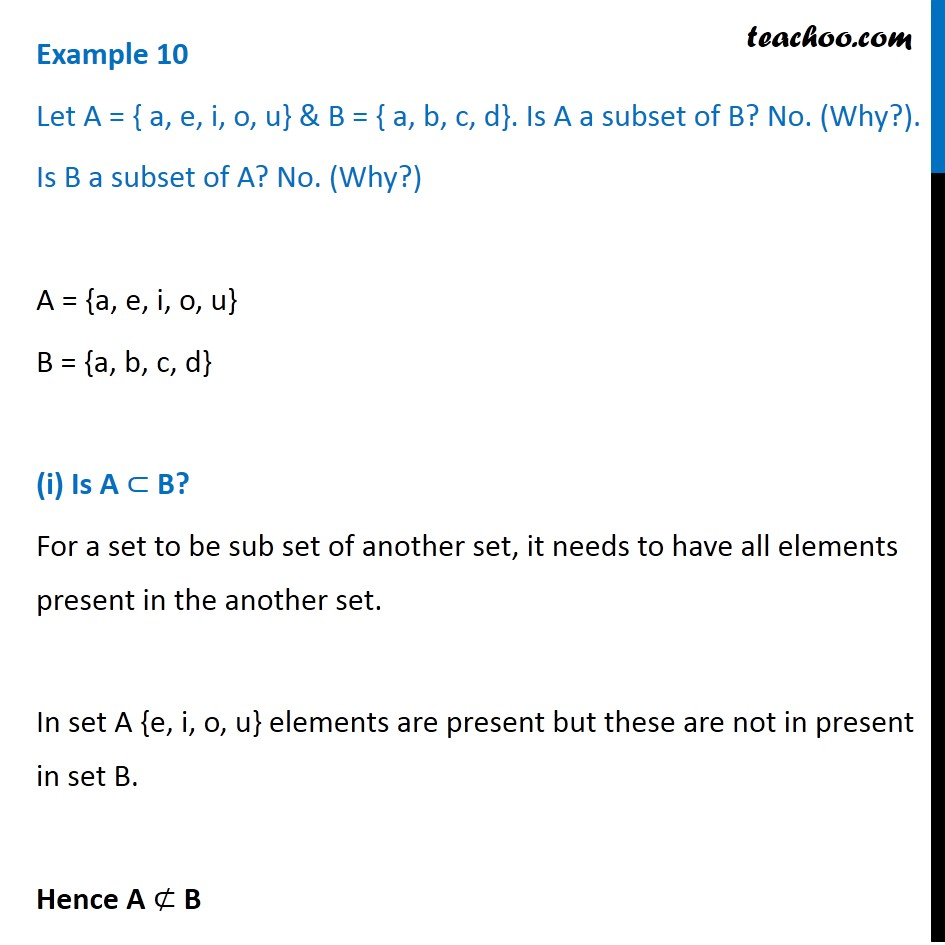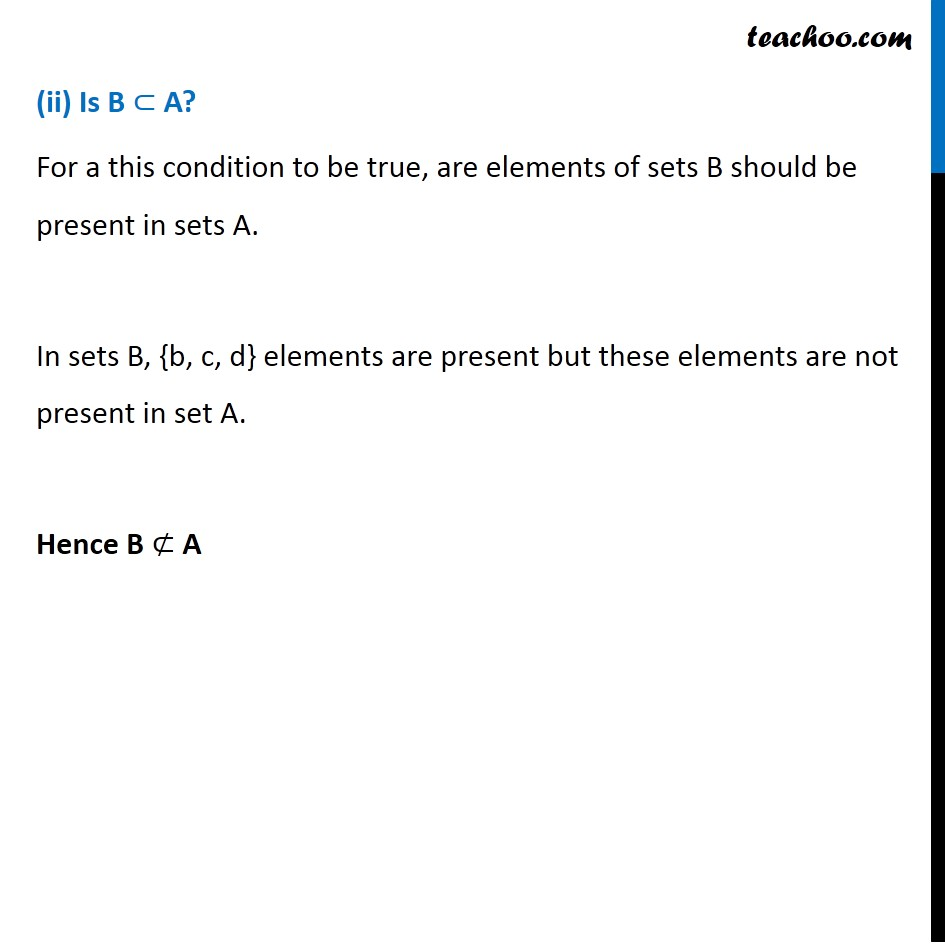Learn about Sets on our Youtube Channel - https://you.tube/Chapter-1-Class-11-Sets1. Chapter 1 Class 11 Sets
2. Concept wise
3. Subset

Transcript

Example 10 Let A = { a, e, i, o, u} & B = { a, b, c, d}. Is A a subset of B? No. (Why?). Is B a subset of A? No. (Why?) A = {a, e, i, o, u} B = {a, b, c, d} (i) Is A ⊂ B? For a set to be sub set of another set, it needs to have all elements present in the another set. In set A {e, i, o, u} elements are present but these are not in present in set B. Hence A ⊄ B (ii) Is B ⊂ A? For a this condition to be true, are elements of sets B should be present in sets A. In sets B, {b, c, d} elements are present but these elements are not present in set A. Hence B ⊄ A

Subset

About the AuthorDavneet Singh
Davneet Singh is a graduate from Indian Institute of Technology, Kanpur. He has been teaching from the past 9 years. He provides courses for Maths and Science at Teachoo.